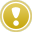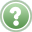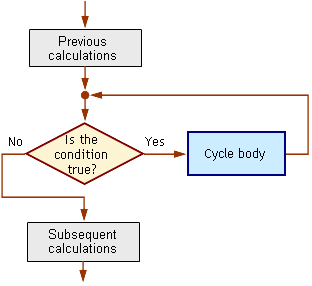English

MQL4 BookOperatorsCycle Operator 'while'

# Cycle Operator 'while'

The most powerful functionality of MQL4 is the possibility to organize cycles (loops).

When creating application programs, you may often use the repeated calculations, which are mostly the repeated program lines. In order to make the programming comfortable and the program itself user-friendly, we use cycle operators. There are two cycle operators in MQL4: while and for. We will consider the first one in this section.

## Format of the Operator 'while'

The full-format cycle operator 'while' consists of the header containing a condition, and the executable cycle body enclosed in braces.

`   while ( Condition )                                  // Header of the cycle operator      {                                            // Opening brace      Block of operators                           // The body of a cycle operator may consist..      that compose the cycle body                  //.. of several operators      }                                            // Closing brace`

If the cycle body consists of only one operator in the operator 'while', you can omit braces.

`   while ( Condition )                                  // Header of the cycle operator      One operator, the cycle body                  // Cycle body is one operator`

## Execution Rule for the Operator 'while'As long as the Condition of the operator 'while' is true: The program passes the control to the operator of the cycle body; after all operators in the cycle body have been executed, it passes the control to the header to test the truth of the Condition. If the Condition of the operator 'while' is false, the control must be passed to the operator that follows the cycle operator 'while'.

Let's consider an example.Problem 12. Calculate the Fibonacci coefficient with the accuracy to 10 significant figures.

First, let's briefly describe Fibonacci coefficient. The Italian mathematician, Leonardo Fibonacci, discovered a unique sequence of numbers:

1 1 2 3 5 8 13 21 34 55 89 144 233 ...

Each number in this sequence is the sum of two preceding numbers. This number sequence has some unique properties: The ratio of two successive numbers in the sequence is equal to 1.618, whereas the ratio between a number to the preceding number is equal to 0.618. Ratio 1.618 was named after Fibonacci, as well as the above sequence is named Fibonacci sequence (it should also be noted that 0.3819, a conjugate for Fibonacci number, was obtained by its multiplying by itself: 0.3819 = 0.618 х 0.618).

The task is to calculate the Fibonacci number with a higher accuracy. If we analyze the Fibonacci coefficient for several tens of the elements of Fibonacci sequence, it becomes obvious that the obtained coefficients range around the irrational number of 1.61803398875..., taking larger or smaller values by turns. The larger numbers of the sequence are involved in the calculations, the smaller is the deviation of the result from this value.

We don't know in advance what exact numbers we should take for their coefficients to differ from each other only after the tenth significant figure. So it is necessary to compose a program that would consecutively search in coefficients until the difference between them makes less than 0.0000000001.

In this case, we created a script (fibonacci.mq4) to solve Problem 12, because the program will not be used for a long time to check every tick. Once attached to the symbol window, the script must perform all necessary calculations (including displayed results), after that it will be unloaded from the window by the client terminal.

`//----------------------------------------------------------------------------------------// fibonacci.mq4// The code should be used for educational purpose only.//----------------------------------------------------------------------------------------int start()                               // Special function start()  {//----------------------------------------------------------------------------------------   int i;                                 // Formal parameter, counter   double   A,B,C,                                 // Numbers in the sequence   Delta,                                 // Real difference between coefficients   D;                                     // Preset accuracy//----------------------------------------------------------------------------------------   A=1;                                   // Initial value   B=1;                                   // Initial value    C=2;                                   // Initial value   D=0.0000000001;                        // Set accuracy   Delta=1000.0;                          // Initial value//----------------------------------------------------------------------------------------   while(Delta > D)                       // Cycle operator header     {                                    // Opening brace of the cycle body      i++;                                // Counter      A=B;                                // Next value      B=C;                                // Next value      C=A + B;                            // Next value      Delta=MathAbs(C/B - B/A);           // Search difference between coefficients     }                                    // Closing brace of the cycle body//----------------------------------------------------------------------------------------   Alert("C=",C," Fibonacci number=",C/B," i=",i);//Display on the screen   return;                                // Exit start()  }//----------------------------------------------------------------------------------------`

At the beginning of the program, variables are declared (and described). In the subsequent lines, numeric values are assigned to the variables. A, B and C take the value of the first numbers in Fibonacci sequence. It must be noted here that, while the sequence itself contains integers only, the quotient of their division must be considered in the program as a real number. If we used the int type for these numbers here, it would be impossible to calculate Fibonacci coefficient, for example: 8/5 = 1 (integer 1, without the fractional part). So, in this case, we use the variables of double type. The cycle operator as such looks like this:

`   while(Delta > D)                       // Cycle operator header     {                                    // Opening brace of the cycle body      i++;                                // Counter      A=B;                                // Next value      B=C;                                // Next value      C=A + B;                            // Next value      Delta=MathAbs(C/B - B/A);           // Search difference between coefficients     }                                    // Closing brace of the cycle body`

Let's consider a block diagram of the cycle operator 'while':Fig. 42. Block diagram of the operator-'while' execution in program fibonacci.mq4.

The cycle operator starts working with testing the condition. The cycle will be performed repeatedly, until the condition (Delta>D) is true. You will understand the program code much better, if you say the key phrase (the execution rule) aloud while reading the operators. For example, if you read the operator 'while' it is: "As long as .., perform the following: ..". In this case, the header of the operator 'while' is as follows: As long as Delta is greater than D, perform the following... Then you can go to analysis of program lines composing the cycle body and being executed if this Condition is true.

Before the control in the above example of fibonacci.mq4 is passed to the cycle operator 'while', the values of these variables are equal to 1000.0 and 0.0000000001, respectively; so the condition is true at the first call to the cycle operator. This means that, after the condition has been tested, the control will be passed to the first operator in the cycle operator body.

In our example, it is the following operator:

`      i++;                                // Counter`

In the three subsequent lines, the values of the next set of sequence elements will be calculated:

`      A = B;                              // Next value      B = C;                              // Next value      C = A + B;                          // Next value`

It's easy to see that the variables take the values of the next (nearest larger) elements. Before the cycle operator is executed, the values of A, B and C have been equal to 1.0, 1.0 and 2.0, respectively. During the first iteration, these variables take the values of 1.0, 2.0 and 3.0, respectively.

Iteration is a repeated execution of some calculations; it is used to note that the program lines composing the cycle operator body are executed.

In the next line, we calculate the difference between Fibonacci numbers obtained on the basis of subsequent (C/B) and preceding (B/A) elements of the sequence:

`      Delta = MathAbs(C/B - B/A);         // Search difference between coefficients`

In this operator, we use the standard function MathAbs() that calculates the absolute value of the expression. As we mentioned above, Fibonacci coefficients take, in turns, larger and smaller values (as compared to the 'standard') with increase of the values of the sequence elements. This is why the difference between neighboring coefficients will take now a negative, now a positive value. At the same time, we are really interested in the value itself of this deviation, i.e., its absolute value. Thus, whatever is the direction the current value of Fibonacci number is deviated in, the value of expression MathAbs(C/B - B/A), as well as the value of variable Delta, is always positive.

This operator is the last in the list of operators composing the cycle body. This is confirmed by the presence of a closing brace in the next line. After the last operator in the cycle body has been executed, the control is passed to the cycle operator header for testing the condition. This is the key point that determines the essence of the cycle operator. According as the cycle operator condition is true or false, the control will be passed to either the next iteration orо outside the cycle operator.

At the first iterations, the value of variable Delta turns out to be greater than the value defined in the variable D. This means that the condition (Delta > D) is true, so the control will be passed to the cycle body for it to perform the next iteration. All variables involved in the calculations will take new values: as soon as the end of the cycle body is reached, the control will be passed to the header again in order to test the condition for being true.

This process will continue, until the condition of the cycle operator becomes false. As soon as the value of the variable Delta becomes less or equal to the value of D, the condition (Delta > D) is not true any longer. This means that the control will be passed outside the cycle operator, to the line:

`   Alert("C=",C," Fibonacci number=",C/B," i=",i);//Display on the screen`

As a result, the Alert() operator window containing the following message will appear on the screen:

 С=317811 Fibonacci number=1.618 i=25

This means that the search accuracy is reached at the 25th iteration, the maximum value of Fibonacci sequence element processed being equal to 317811. Fibonacci coefficient, as expected, is equal to 1.618. This message is the solution of the stated problem.

It must be noted here that real numbers are calculated in MQL4 with an accuracy to 15 significant figures. At the same time, Fibonacci coefficient is displayed with an accuracy to 3 significant figures. This is determined by the fact that the function Alert() displays numbers with an accuracy to 4 significant figures, without displaying the zeros at the end of the number. If we wanted to display Fibonacci number with a certain predefined accuracy, we would have to change the code, for example, in this way:

`   Alert("C=",C," Fibonacci number=",C/B*10000000," i=",i);// Message`

The following must be particularly noted:It is possible that the condition specified in the cycle operator header always remains true. This results in looping.

Looping is a continuously repeated execution of operators composing the cycle body; it's a critical situation that results from realization of a wrong algorithm.

Once a looping takes place, the program endlessly executes the block of operators composing the cycle body. Below is a simple example of a looped cycle operator 'while':

`   int i=1;                               // Formal parameter (counter)   while (i > 0)                          // Cycle operator header      i++;                                // Increment of the value of i`

In the above example, the values of variable i are accumulated (incremented) at every iteration. As a result, the condition will never become false. A similar situation occurs, if nothing is calculated in the cycle body at all. For example, in the code below:

`   int i=1;                               // Formal parameter (counter)   while (i > 0)                          // Cycle operator header      Alert("i= ",i);                     // Display on the screen`

the value of variable i in the cycle does not change. The following message will be shown in the screen at each iteration:

 i= 1

This process will repeat endlessly. Once having got into the trap of a looped code, the control cannot leave it anymore. This situation is particularly dangerous in trading Expert Advisors and scripts. In such cases, the environment variables are not usually updated, since the special function does not complete its operation, whereas the trader may be unaware of the existing looping. As a result, the control in the executing program cannot be passed to the corresponding program lines where the decision of opening or closing orders is made.

The programmer must prevent such conditions, in which an uncontrolled looping becomes possible. There is no technique that would help to discover this situation programmatically neither at compiling of the program nor at its execution. The only possible method to detect such algorithmic errors is close examination of your code - logical reasoning and common sense.Conditional Operator 'if - else'Cycle Operator 'for'